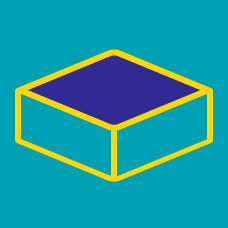Geometry

# Volume - Cuboid

The swimming pool that Calvin goes to is a rectangular prism that has dimensions 20 meters long, 8 meters wide and 3 meters deep. What is the volume of water that the swimming pool contains (in $\mbox{m}^3$)?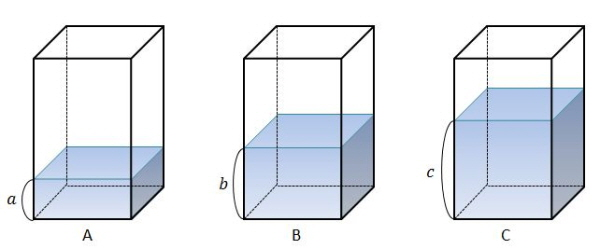In the above diagram, three identical containers $A,$ $B$ and $C$ contain water reaching heights $a,$ $b$ and $c,$ respectively. If the respective water volumes are $12\text{ cm}^3,$ $20\text{ cm}^3$ and $28\text{ cm}^3,$ what is the ratio $a:b:c ?$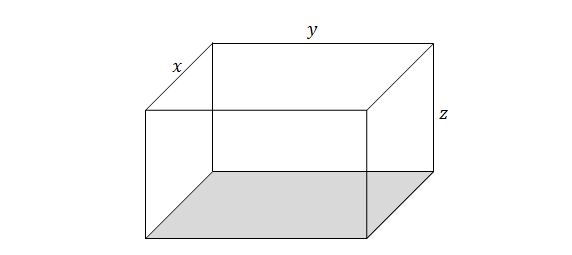The above diagram is a rectangular solid with width $x,$ length $y$ and height $z.$ If $xy=10,$ $yz=20$ and $zx=8,$ what is the volume of the rectangular solid?

Note: The above diagram is not drawn to scale.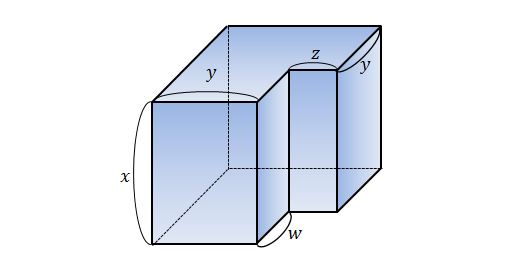In the above diagram, a small rectangular cuboid is cut off from a larger rectangular cuboid. If $x=7,$ $y=4,$ $z=2$ and $w=3,$ what is the volume of remaining body?

Note: The above diagram is not drawn to scale.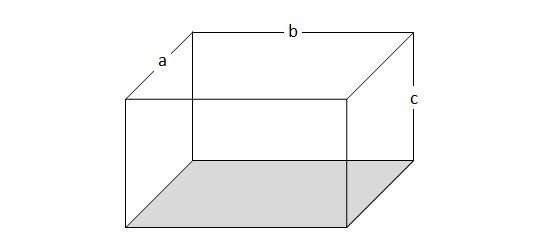If $a=9,$ $b=14$ and $c=9$ in the above diagram, what is the volume of the rectangular cuboid?

×